# How to calculate (rather than format) a percentage in Excel

AUTHOR:  JASON MORRELLminutes remainingMaster Microsoft Office
in 30 days or less

YOU MAY ALSO LIKE

Many people can’t do percentages.

Can't get their head around them.

Can't do the maths.

So putting together an Excel percentage formula seems impossible!

Have you ever asked yourself any of these questions?

• “How do I write percentage formulas?”
• “How do I calculate percentages?”
• “How do I increase x by y percent?”
• “How do I add x% to y?”
• “How do I subtract x% from y?”

These are some of the most common questions and challenges that people have. I get asked so today I have simplified it and laid it all out in a post for you. You're welcome!

## 1.  Percentages vs Numbers

The first thing to understand is the correlation between percentages and numbers. Think of it like this: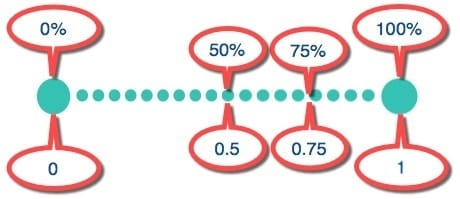Figure 01: Percentage sliding scale

Imagine a scale that goes from 0 to 1, where 0 = 0% (i.e. nothing) and 1 = 100% (i.e. everything).

Now travel half way along that scale and you have 0.5 or 50%.

Travel three quarters of the way along the scale and you have 0.75 or 75%.

So the relationship can be expressed like this:

the percentage = the decimal number multiplied by 100,
with a % symbol added to the end.

Try this.

1.       Enter 0.5 into a cell and press Enter.

2.      Re-select the cell

3.      Click the % icon in the Number group on the Home ribbon. This converts the decimal number to a percentage.

4.      Click the comma style icon (next to the % button) to convert back to a number. The formatting defaults to 2 decimal places. You can adjust this by clicking the increase/decrease decimal places icons.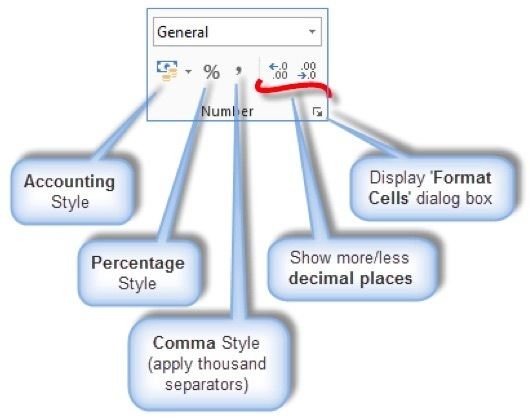Figure 02: Formatting icons at your fingertips on the Home tab

## 2. Common mistake: Formatting percentages rather than calculating percentages

Consider the following range of numbers: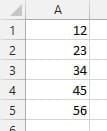Figure 03: Starting values

What is the percentage of each number?

If you select the range of cells and click the % button mentioned above, you're in for a surprise!

Excel is doing exactly what it is supposed to to. It has multiplied each number by 100 and added the % symbol on the end.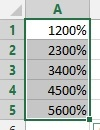Figure 04: Shock! Horror!

## 3. How to calculate percentages the proper way

The original question - ‘What is the percentage of each number?’ - doesn’t actually make sense.

The correct question is - ‘What percentage is each number of the total?’

Try this:

1.       Select cells A1:A5, if necessary.

2.      Click the comma style icon to change the formatting back to ordinary numbers.

3.      Decrease the decimal places until you are showing whole numbers again.

4.      Select cell A6.

5.      Click the AutoSum button on the right side of the Home ribbon, to calculate a total.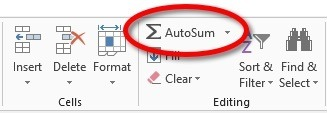Figure 05: The AutoSum Button

6.      In cell B1, type the formula: =A1/A6.

7.      Before you press Enter, press the F4 key to make the call reference absolute (A6 becomes \$A\$6 which means it is now a fixed reference).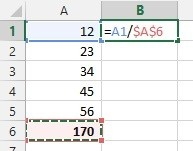Figure 06: Percentage formula with absolute cell reference

8.      Press Enter.

9.      Re-select cell B1.

10.    Grab the auto fill handle (the small block on the bottom right corner of the cell) and double-left-click. This will copy the formula down to cell B5. You now have 5 decimal results.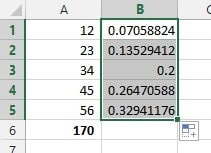Figure 07: Results currently showing as decimal figures

11.      Select cells B1:B5, if necessary.

12.     Click the % icon in the Number group on the Home tab, then increase the decimal places to show all figures to 1 decimal place.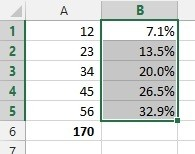Figure 08: Results now displayed as percentages

13.      Select cell B6.

14.     Click the AutoSum icon. You will see that the total of all the percentages is 100%.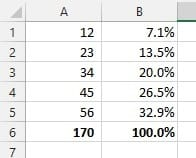Figure 09: Individual percentage figures will total 100 percent

### a) Example #1: How to add 20% Sales Tax

Or, to put it another way - How to increase a figure by 20%.

Most countries have some form of sales tax. In the UK , its called VAT (Value Added Tax). In Australia it’s called GST (General Services Tax). In the US it’s just called Sales Tax.

Excel doesn’t care what you call it. It just sees a percentage!

So how do you add a percentage of sales tax to a base figure to reach a total?

Try this on a fresh sheet:

1.       In cell A1, type your base figure (e.g. 1,000). NB. Don’t type currency symbols or commas, just the plain number.

2.      In cell B1, type the sales tax percentage (e.g. 20%).

3.      In cell C1, type the formula: =A1*B1. This calculates the amount of sales tax.

4.      In cell D1, type the formula: =SUM(A1, C1). This is your total.

To combine steps 3 and 4 into a single step:

In cell E1, type the formula:

###### =SUM(A1,A1*B1)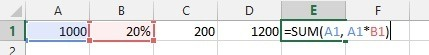Figure 10: Adding sales tax to a base figure to calculate a total

### b) Example #2:Using percentages to remove sales tax from a total

Let’s say that you have a total figure of \$1,200 which comprises \$1,000 plus \$200 sales tax. How do get back to the base figure of \$1,000.

The maths goes like this: Divide the total figure by 120% (assuming a sales tax of 20%).

It’s bad practice to use fixed figures like 120% so here’s how to calculate it using a formula that makes use of the 20% cell.

E1 is the total sales figure.

B1 is the sales tax rate percentage.

The formula to remove the 20% sales tax from the total sales figure is ...

This equates to

### c) Example #3: Using percentages to calculate interest on a loan

If cell A1 contains a loan amount, let’s say 200,000,
cell A2 contains an interest percentage, let’s say 5% (per annum) ...

... you simply multiply one by the other to calculate the amount of interest due per annum, i.e.

## 4. Watch the video (over the shoulder demo)## 5. What next?

I hope you’ve found this useful. It’s surprising how many people struggle with percentages and I hope I’ve simplified the process for you.

What do you think?

Please feel free to post any questions you have into the comments below. And before you leave this page, I want to give you one quick little action item!

ACTION ITEM: Comment below with ONE thing you picked up from this post and share how it has helped, or will help, you. It's good to share your successes because it encourages others and provides an instant boost.

Enjoy the rest of your day!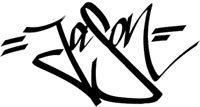Jason Morrell

Jason loves to simplify the hard stuff, cut the fluff and share what actually works. Things that make a difference. Things that slash hours from your daily work tasks. He runs a software training business in Queensland, Australia, lives on the Gold Coast with his wife and 4 kids and often talks about himself in the third person!

SHARE

``` (adsbygoogle = window.adsbygoogle || []).push({}); ```
``` (adsbygoogle = window.adsbygoogle || []).push({}); ```

Check the RELATED articles below

## 2 Ways to Build a Dynamic Microsoft Word Index Page

2 Ways to Build a Dynamic Microsoft Word Index Page

## Multilevel numbering in Word that works perfectly, first time

Multilevel numbering in Word that works perfectly, first time

## How many rows and columns does Excel have?

How many rows and columns does Excel have?